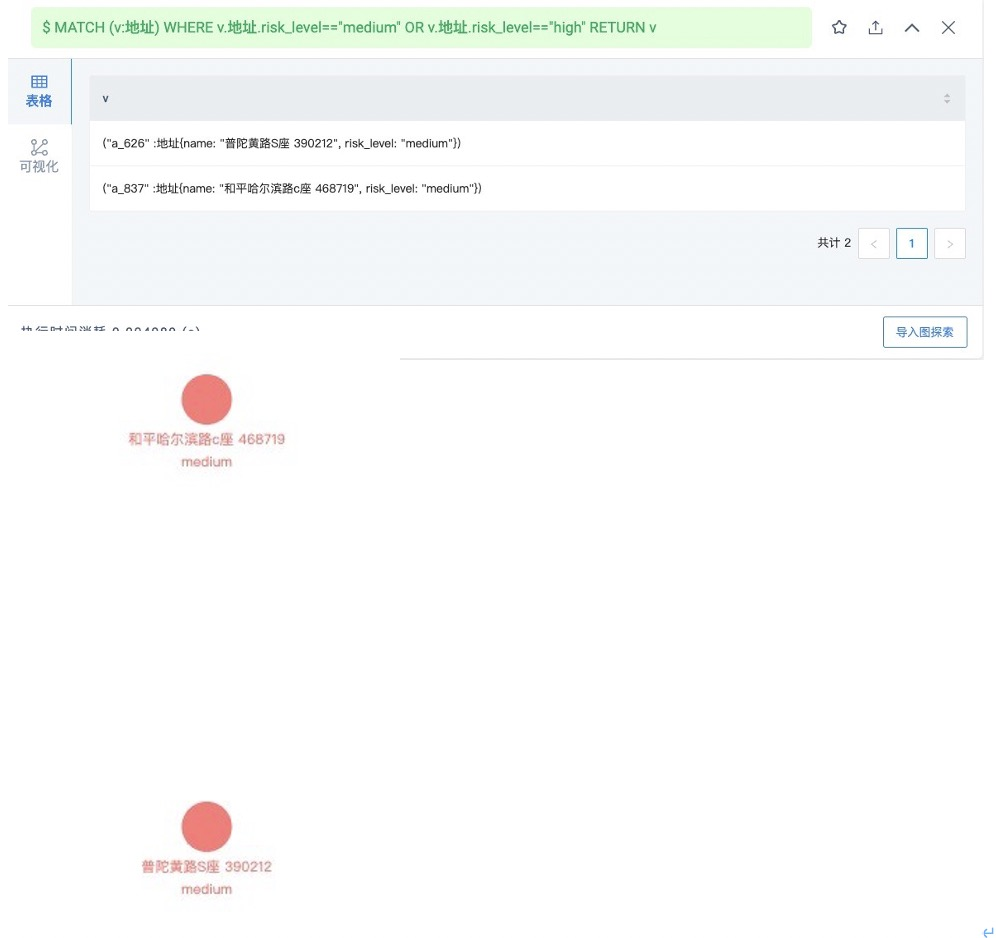# Demo 介绍｜Covid Tracing 疫情防控

## 快速开始：

1. 从这里访问 悦数 Playground: covid_trace

2. 选择 ovid_trace 图空间，点击中间的“快速开始（随机导入数据）”，点击“Query by VID”

3. 这个时候在画布上已经显示出点了，您可以双击他们进行拓展，或者借助左侧功能栏，进行其他操作

## 操作步骤

1. 查看所有人员核酸检测结果概况2.新确诊一名阳性患者，更新健康信息，了解个人信息

1）随机挑选一位正常人员，更新健康信息为阳性，并查看个人信息

MATCH (p:人) WHERE p.人.is_confirmed==false RETURN p LIMIT 1;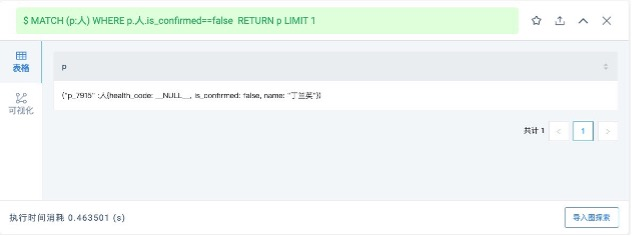2）上传确诊患者信息

UPDATE VERTEX ON 人 "p_7915" SET is_confirmed=true YIELD is_confirmed AS Infected; INSERT VERTEX 联系方式(num) VALUES "13312341234":(13312341234); INSERT EDGE 住址() VALUES "p_7915"->"a_626";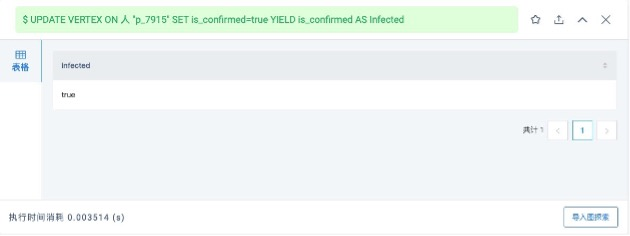3）查询该患者家庭住址、联系方式等信息

MATCH p=(v:人)-[e:住址|属于]-(v2) WHERE id(v)=="p_7915" RETURN p;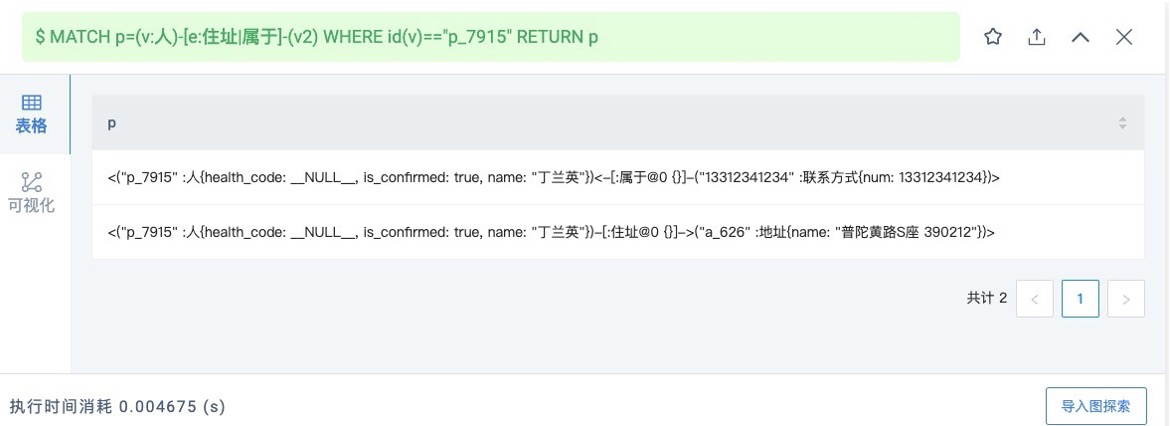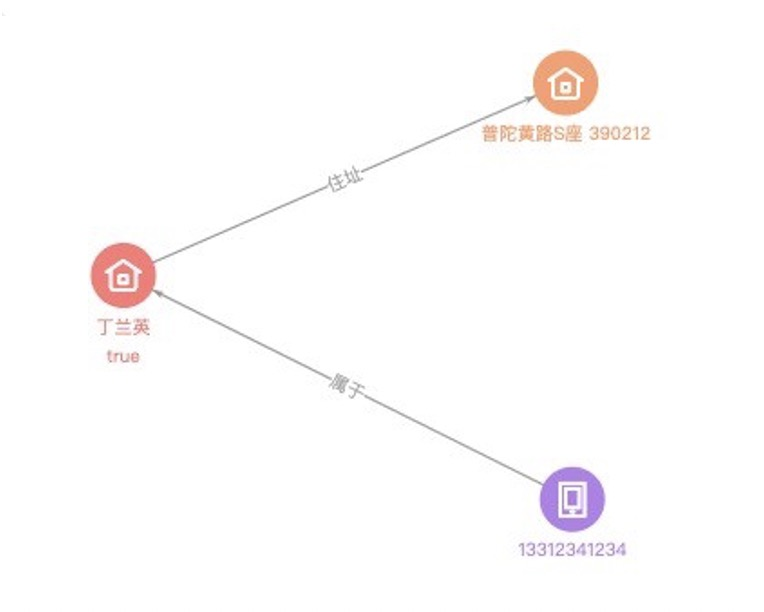3.查找其同住人、时空伴随者(场所码) 等密接

1）查找同住人（密接）

MATCH p=(v:人)-[e:同住]-(v2) WHERE id(v)=="p_7915" RETURN p;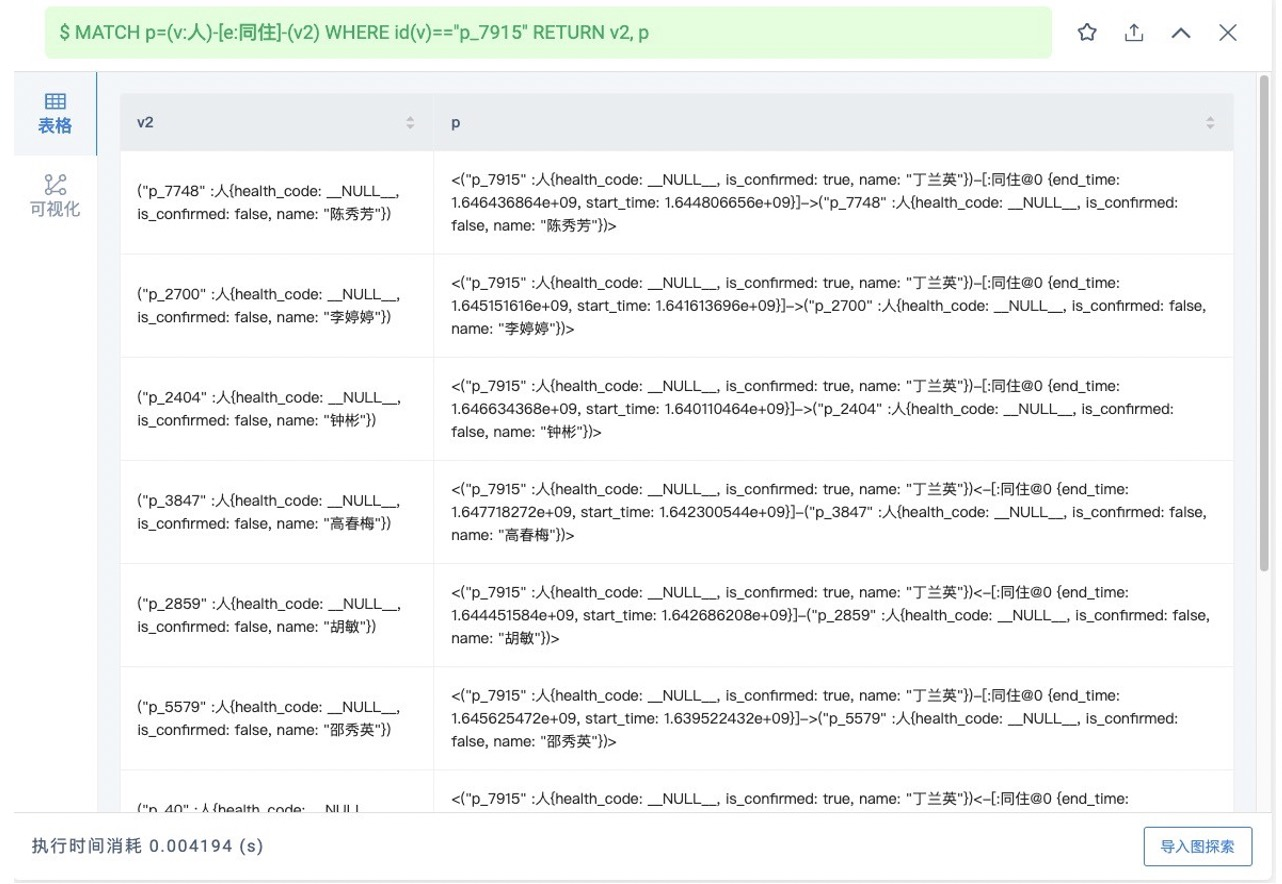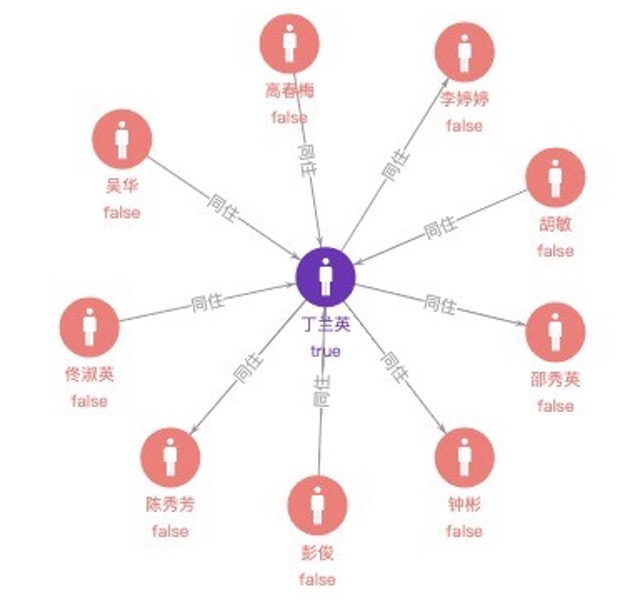2）查找其直接时空伴随者（密接）

MATCH p=(v:人{name:"丁兰英"})-[e:到访]->(v1:地址)<-[e1:到访]-(v2:人) WHERE e.start_time < e1.start_time AND e.end_time > e1.end_time RETURN p;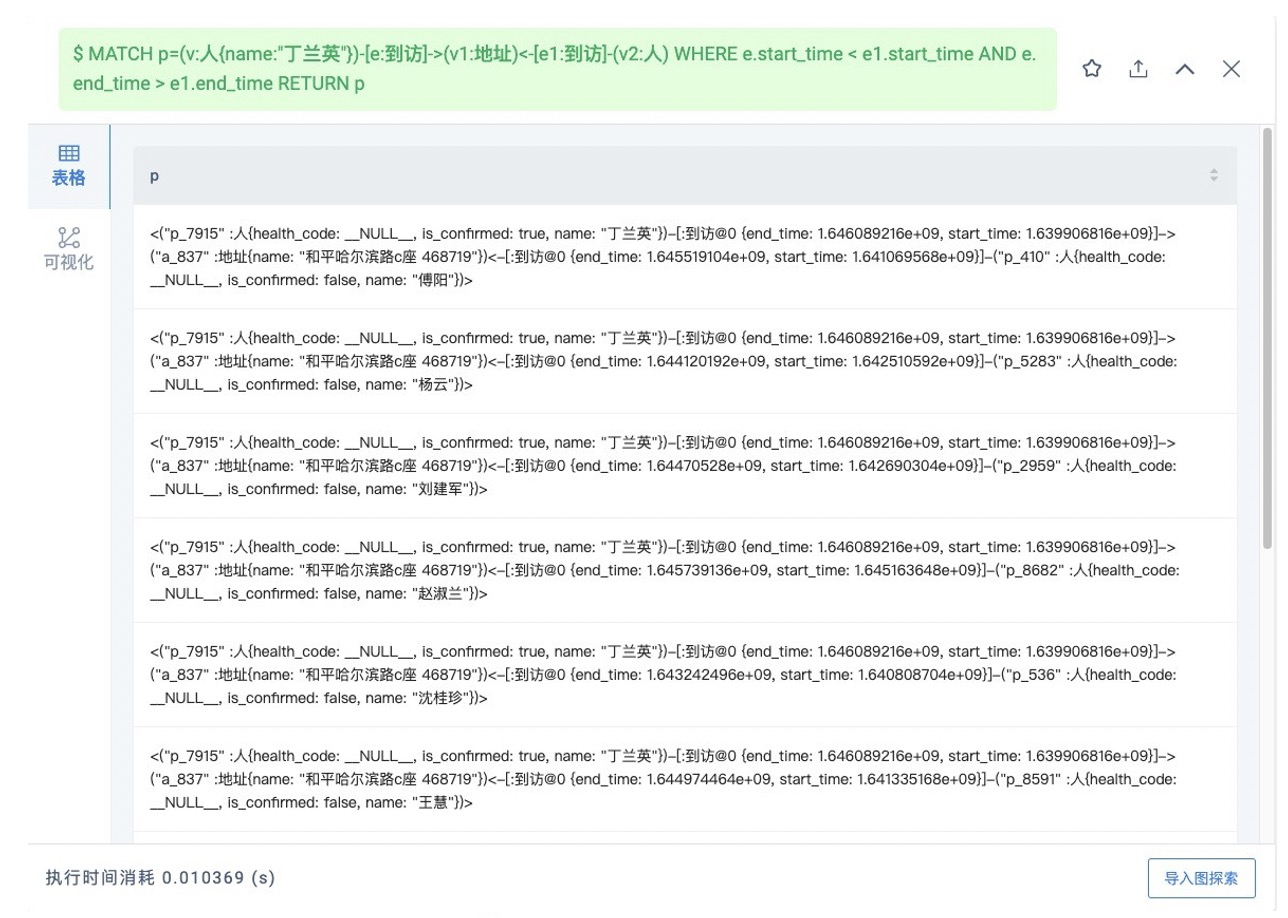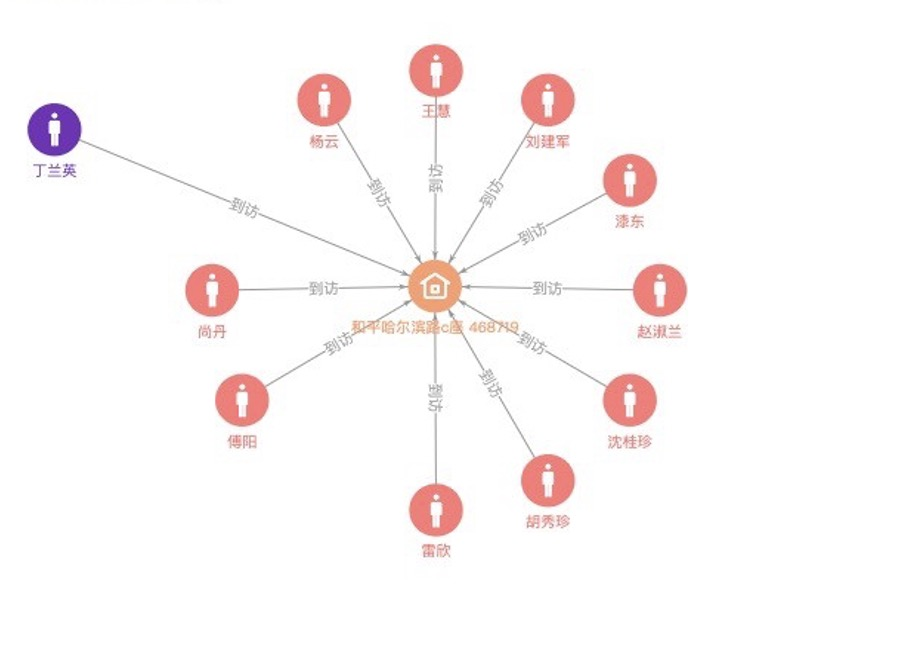4.查找其所有的次密接

1）同住人导致的次密接数及路径

MATCH p=(v:人{name:"丁兰英"})-[e:同住]-(v1:人)-[e1:到访]->(v2:地址)<-[e2:到访]- (v3:人) WHERE e1.start_time < e2.start_time AND e1.end_time > e2.end_time RETURN COUNT(DISTINCT v3);

MATCH p=(v:人{name:"丁兰英"})-[e:同住]-(v1:人)-[e1:到访]->(v2:地址)<-[e2:到访]- (v3:人) WHERE e1.start_time < e2.start_time AND e1.end_time > e2.end_time RETURN p;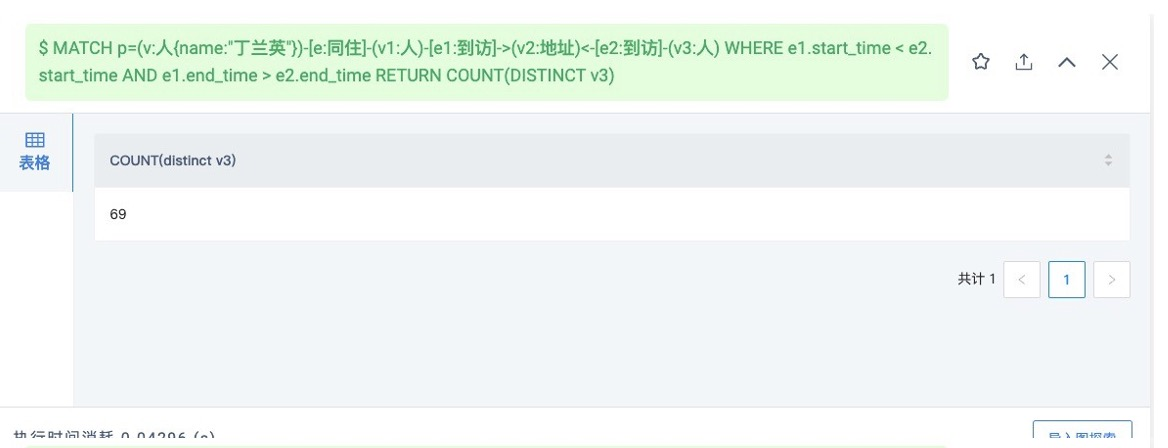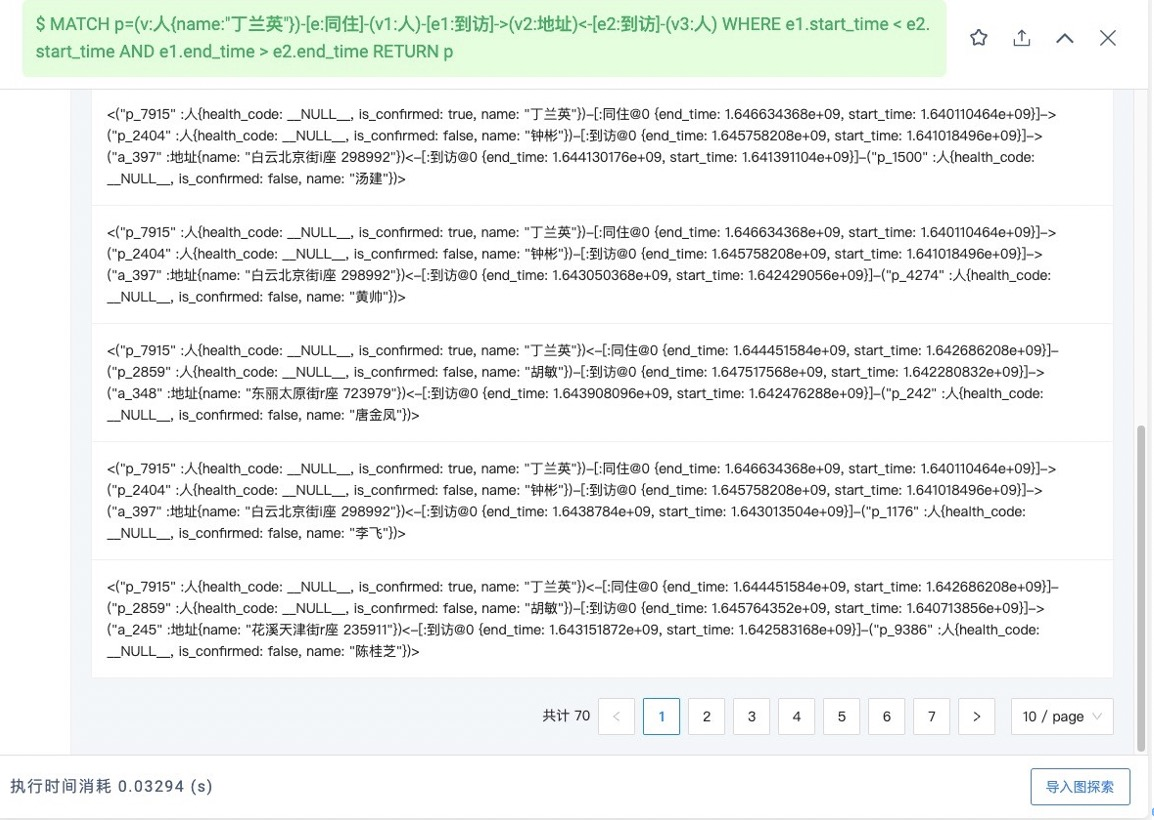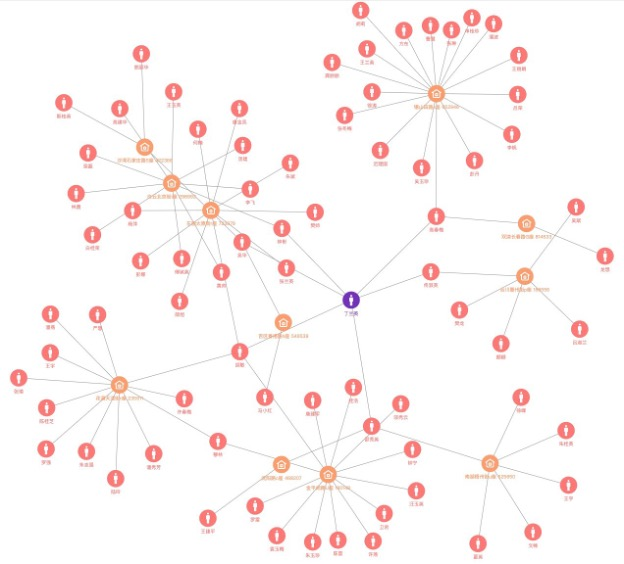2）其它时空伴随导致的次密接数及路径

MATCH p=(v:人{name:"丁兰英"})-[e:到访]->(v1:地址)<-[e1:到访]-(v2:人)-[e2:到访]->(v3:地址)<-[e3:到访]-(v4:人) WHERE e.start_time < e1.start_time AND e.end_time > e1.end_time AND e2.start_time < e3.start_time AND e2.end_time > e3.end_time RETURN v2, v4, p;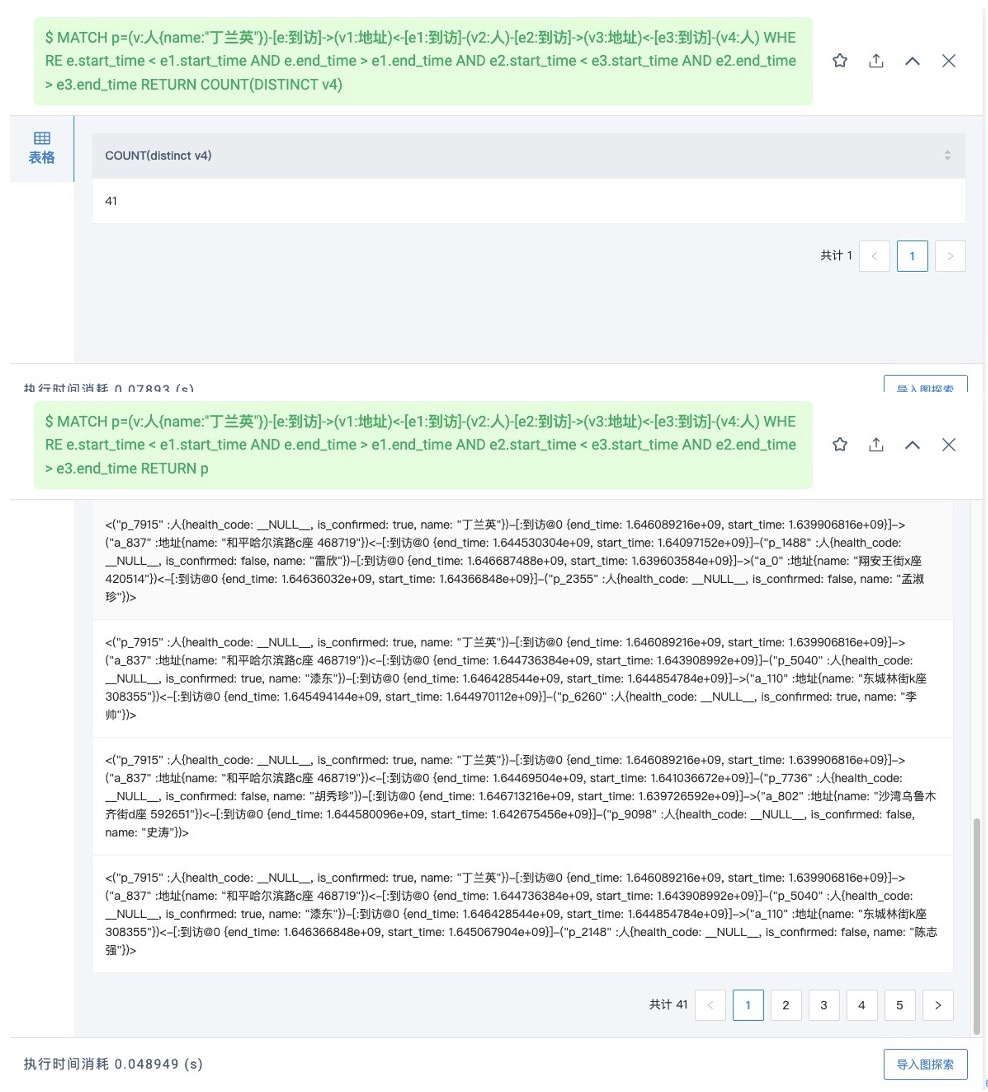5.密接管控人中陆续检测出阳性，上传更新相关数据，查询该条传播链情况

UPDATE VERTEX ON 人 "p_7748" SET is_confirmed=true YIELD is_confirmed AS Infected;

UPDATE VERTEX ON 人 "p_2700" SET is_confirmed=true YIELD is_confirmed AS Infected;

UPDATE VERTEX ON 人 "p_5040" SET is_confirmed=true YIELD is_confirmed AS Infected;

UPDATE VERTEX ON 人 "p_6260" SET is_confirmed=true YIELD is_confirmed AS Infected;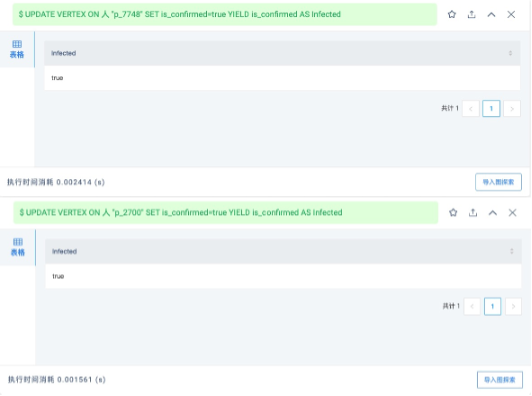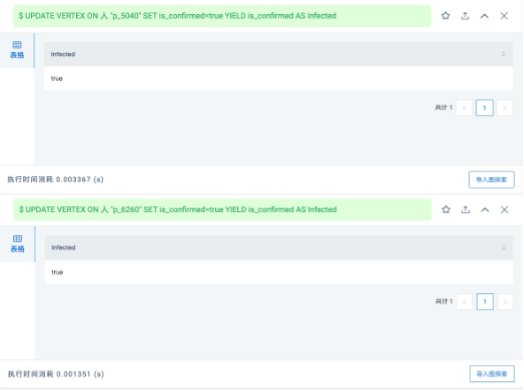6.统计密接中阳性数，如果有则表明形成了传播，需要将应对场所标记为风险地区

1）同住人中阳性数

MATCH p=(v:人{name:"丁兰英"})-[e:同住]-(v1:人) WHERE v1.人.is_confirmed==true RETURN COUNT(v1);

2）其它时空伴随者中的阳性数

MATCH p=(v:人{name:"丁兰英"})-[e:到访]->(v1:地址)<-[e1:到访]-(v2:人) WHERE e.start_time < e1.start_time AND e.end_time > e1.end_time AND v2. 人.is_confirmed==true RETURN COUNT(v2);

3）源头阳性住址置为中风险

UPDATE VERTEX ON 地址 "a_626" SET risk_level="medium" yield risk_level AS Risk;

4）到访地置为中风险

UPDATE VERTEX ON 地址 "a_837" SET risk_level="medium" yield risk_level AS Risk;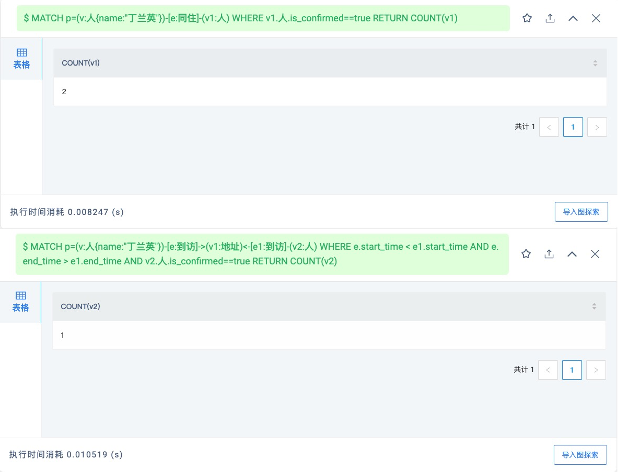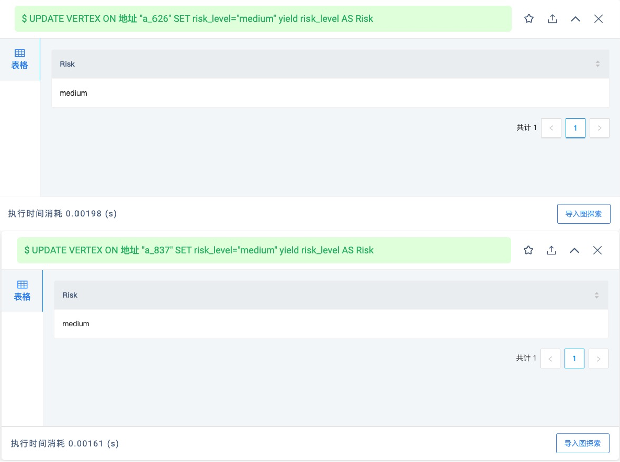7.查看当前中风险地区

MATCH (v:地址) WHERE v.地址.risk_level=="medium" OR v.地址.risk_level=="high" RETURN v;